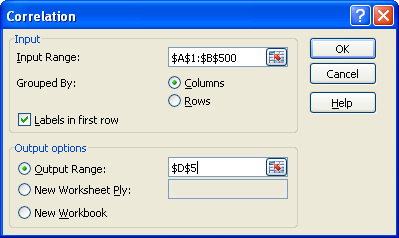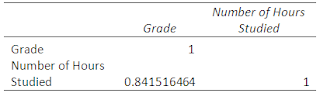# Correlation Matrix Using Excel

This article explains how to compute a correlation matrix Using MS Excel. With the help of Analysis ToolPak, a correlation matrix can be generated quickly and easily.

Correlation is used to measure strength of the relationship between two variables. It can be positive, negative or zero.

Positive Correlation : Both variables tend to move in the same direction: If one variable increases, the other tends to increase. If one decreases, the other tends to decrease.

Negative Correlation : Both variables tend to move in the opposite directions: If one variable increases, the other tends to decrease, and vice-versa.

Note: The correlation coefficient may take on any value between +1 and -1.

Examples
1: How are sales of ABC Company and GDP related?
2: How are annual returns on Treasury Bills and Bonds related?
What is correlation matrix?

When you need to calculate correlation between more than two variables, it is generally called correlation matrix. It is also called multiple correlation coefficient. Let's solve it with a case study.

Suppose you would like to know whether there is a relationship between grades and number of hours you spend studying.
You can download the workbook used in this example and practice what you learn.

Steps to calculate Correlation Matrix in Excel
1. If you haven't already installed the Analysis ToolPak, click the Microsoft Office button, then click on Excel Options, and then select Add-Ins. Click Go, check the Analysis ToolPak box, and click OK. For more details on how to install the Analysis ToolPak, refer to the How to Install Analysis ToolPak.
2. Select the Data tab, then click on the Data Analysis option, and choose Correlation from the list. Click OK. (Data tab >> Data Analysis >> Correlation)
3. Select the data range (both independent and dependent variables) in the Input Range box.
4. Check the Labels in first row option, enter the range in the Output Range box, and click OK.Excel : Multiple Correlation CoefficientOutput : Correlation Coefficient

Interpretation:
As you see the correlation between the grades and number of hours you spend studying is a very positive correlation (84%). This means as more number of hours students study their grades improve.Understand Correlation

Calculate correlation coefficient with CORREL function

By using CORREL function, you can calculate correlation coefficient of two variables (ranges)

Syntax:
=CORREL (range1, range2)

For the above problem the syntax would be =CORREL(\$A\$2:\$A\$500, \$B\$2:\$B\$500)

To calculate multiple correlation coefficient with Excel Formula, visit this link : Formula Explained:Correlation Matrix

How to deal with outliers when doing correlation?
Solution: Look at the residuals from a regression by plotting the points to a scatter diagram. If they are not normally distributed around 0 the realibility of the Pearson correlation could be unreliable.

Fun : In a heated argument, the regression shouted at correlation, "You are dumbass, you don’t even know the cause and effect of this argument"

Related Posts
Share1.